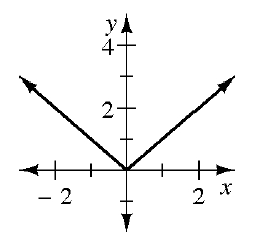### Home > INT2 > Chapter 9 > Lesson 9.3.3 > Problem9-107

9-107.

The absolute value function $f(x)=\left|x\right|$ is sometimes written as a piecewise-defined function.

1. Sketch a graph of $f(x)=\left|x\right|$.1. Write a set of equations to represent $f(x)$ as a piecewise-defined function. Be sure to include the domain for each.

For $x\ge0$, what is the equation of the ray?

For $x<0$, what is the equation of the ray.

$f(x) = \left\{ \begin{array}{lr} x & \text{if } x \geq 0 \\ {?} & \text{if } x < 0 \end{array} \right.$